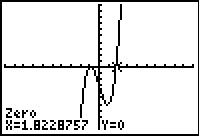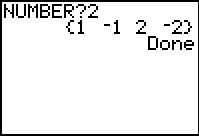# Activities

•##### Download

•• ##### Subject Area

• Math: Precalculus: Complex Numbers
• Math: Precalculus: Polynomial, Power, and Rational Functions

• ##### Author9-12

15 Minutes

• ##### Device
• TI-83 Plus Family
• TI-84 Plus
• TI-84 Plus Silver Edition
•TI-84 Plus C Silver Edition
•TI-84 Plus CE
• ##### Software

TI Connect™
TI Connect™ CE

• ##### Report an Issue

It's a Rat Race for the Zeros#### Activity Overview

Students explore the Rational Root Theorem by comparing sets of functions that have at least one real zero or have no real roots.

#### Key Steps

•In this introductory activity, students will explore the rational root theorem using a program that will output a specific list of factors for an imputed number. First, students will graph two sets of functions and use the zero command to calculate the zeros. They will try to determine what the graphs of the each set of functions have in common.

•Then students will determine how the sets relate to the function and then determine what combination, if any, of the values in the sets will produce a zero of the function. They will explore both sets of functions using the program and the Home screen. After the exploration, the teacher will lead a formal discussion on the Rational Root Theorem.